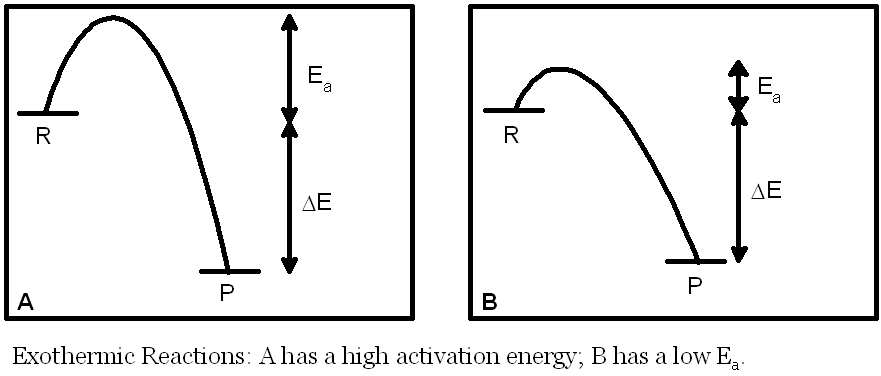## Thermodynamic vs. Kinetic Stability

In the diagrams on this page R stands for reactants, P stands for products. Ea is the activation energy of a reaction (the minimum energy a molecule must have in order to react). ΔE is the overall energy change in the reaction.

It is a universal law that all things seek the lowest energy state. A ball rolls down, not up, a hill. So when a substance is at lower energy, it is more stable. The ball at the bottom of the hill is likely to stay there and not roll back up again. Thermodynamic stability has to do with the relative energies of reactants and products. This stability is independent of the pathway between those reactants and products. Thermodynamic stability can be conceptualized in terms of the chemical potential energy. Chemical potential energy is the energy that can be released from a chemical upon reaction with another chemical. It is usually measured relative to a standard as in the standard enthalpy of formation used in the introduction to chemical thermodynamics. Stable chemicals have low energy: they are not reactive and have low chemical potential energy. Unstable chemicals have high energy: under the right conditions they can release their high chemical potential energy.

Kinetic stability has to do with the pathway between reactants and products. Kinetic stability is largely dependent on the activation energy for the reaction. The activation energy is determined by the chemical potential energy of the reaction intermediate(s). If the activation energy is high then at low temperatures the reaction will be slow. It can be made faster by increasing the temperature. If the activation energy is low then the reaction will be fast even at low temperatures. Such reactions do increase their rates with increasing temperature as well.

Take the ball on the hill again. If the ball is in a depression at the top of the hill then it won’t roll down. It is kinetically stable and needs an input of energy to go uphill before it can roll down to the bottom of the hill. This input of energy is the activation energy.

In summary, thermodynamic stability determines the equilibrium constant (K) and kinetic stability determines the rate constant (k). They are independent of one another. The relative stability of reactants and products is the same regardless of the reaction pathway or mechanism. The activation energy (Ea) depends on the reaction pathway and the specific intermediate(s) involved.

### Exothermic ReactionsIn exothermic reactions the products are more thermodynamically stable than the reactants. The equilibrium constant for the reaction will be large (K>1) for exothermic reactions because ΔE is negative. This has no effect on the rate of the reaction. The rate depends on the activation energy (Ea). For example, reaction A above has a large Ea and therefore would be expected to have a small value for the rate constant for a given temperature (and therefore a slow rate). Reaction B, by contrast, has a smaller Ea and, all other things being equal, therefore would be expected to have a larger value for the rate constant for a given temperature (and therefore a faster rate).

It is possible to imagine a situation in which the two reactions have the same equilibrium constant but reaction A has a slow rate (low rate constant) and reaction B has a fast rate (high rate constant).

page break
Ā

### Endothermic ReactionsIn endothermic reactions the reactants are more thermodynamically stable than the products. The equilibrium constant for the reaction will be small (K<1) for endothermic reactions because ΔE is positive. This has no effect on the rate of the reaction. The rate depends on the activation energy (Ea). For example, reaction A above has a large Ea and therefore would be expected to have a small value for the rate constant for a given temperature (and therefore a slow rate). Reaction B, by contrast, has a smaller Ea and, all other things being equal, a larger value for the rate constant for a given temperature (and therefore a faster rate).

It is possible to imagine a situation in which the two reactions have the same equilibrium constant but reaction A has a slow rate (low rate constant) and reaction B has a fast rate (high rate constant).

### Equilibrium: Reversible Reactions

Notice that the reaction progress diagrams above for each reaction A are the exact reverse of one another. Similarly, both reaction B diagrams are the reverse of one another.

For the reaction A pair the reactions are kinetically slow and so equilibrium would take a long time to achieve. Once it is achieved the lower-energy products (taking the exothermic reaction as the forward reaction) would dominate over the higher-energy reactants.

For the reaction B pair the reactions are kinetically faster and so equilibrium would be achieved relatively quickly. Once it is achieved the lower-energy products (taking the exothermic reaction as the forward reaction) would dominate over the higher-energy reactants (just as for the reaction A pair).

Last updated: Mar 10, 2010       Home